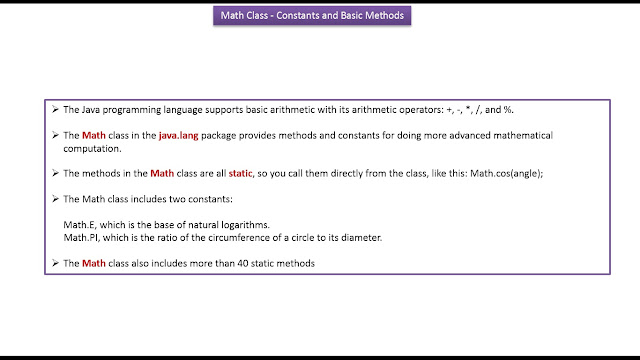Monday, 22 February 2016

Java Tutorial : Java Math class (Constants and Basic methods)

Click the below Image to EnlargeJava Tutorial : Java Math class (Constants and Basic methods)Java Tutorial : Java Math class (Constants and Basic methods)
MathDemo.java
public class MathDemo
{

public static void main(String[] args)
{

double a = -200.635;
double b = 23.74;
int c = 46, d = 65;

/*
* Returns the absolute value of the argument.
*/
System.out.printf(
"The absolute value " + "of %.3f is %.3f%n", a,
Math.abs(a));

/*
* Returns the smallest integer that is greater than
* or equal to the argument. Returned as a double.
*/
System.out.printf("The ceiling of " + "%.2f is %.0f%n", b,
Math.ceil(b));

/*
* Returns the largest integer that is less than or
* equal to the argument. Returned as a double.
*/
System.out.printf("The floor of " + "%.2f is %.0f%n", b,
Math.floor(b));

/*
* Returns the integer that is closest in value to
* the argument. Returned as a double.
*/
System.out.printf("The rint of %.2f " + "is %.0f%n", b,
Math.rint(b));

/*
* Returns the larger of the two arguments.
*/
System.out.printf("The max of %d and " + "%d is %d%n", c, d,
Math.max(c, d));

/*
* Returns the smaller of the two arguments.
*/
System.out.printf("The min of of %d " + "and %d is %d%n", c,
d, Math.min(c, d));
}
}
Output
The absolute value of -200.635 is 200.635
The ceiling of 23.74 is 24
The floor of 23.74 is 23
The rint of 23.74 is 24
The max of 46 and 65 is 65
The min of of 46 and 65 is 46